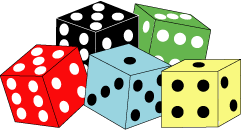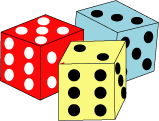#### You may also like### Pebbles

Place four pebbles on the sand in the form of a square. Keep adding as few pebbles as necessary to double the area. How many extra pebbles are added each time?### It Figures

Suppose we allow ourselves to use three numbers less than 10 and multiply them together. How many different products can you find? How do you know you've got them all?### Bracelets

Investigate the different shaped bracelets you could make from 18 different spherical beads. How do they compare if you use 24 beads?

# Scoring with Dice

##### Age 7 to 11Challenge Level

I have a handful of dice:I throw three of them and get 5, 3 and 2:Add the scores on the three dice. What do you get? Now multiply the scores. What do you get?

The next time I throw four of the dice and add and multiply the score as before.
This time the product is exactly 4 times the sum. What scores could I have thrown?

Again I throw four of the dice, adding and multiplying the scores as above. This time the product is exactly 5 times the sum. What scores could I have thrown?

For the last go I throw five of the dice and add and multiply the scores. The product is exactly 6 times the sum. What scores could I have thrown this time?